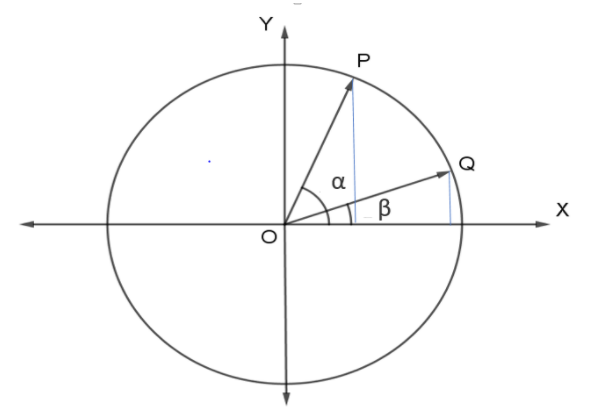QuestionAnswers

# Prove by using vector$\cos \left( {\alpha - \beta } \right) = \cos \alpha \cos \beta + \sin \alpha \sin \beta$Hint: It is sometimes useful to write a vector $v$ in terms of its magnitude and argument rather than rectangular form $a\widehat i + b\widehat j$. This is done using the sine and cosine functions. The $r(\cos \alpha \widehat i + \sin \alpha \widehat j)$ version of $v$ is called trigonometric form. Since $\,\left| {\cos \alpha \widehat i + \sin \alpha \widehat j} \right| = 1$ the trigonometric form expresses $v$ as a scalar multiple of a unit vector in the same direction as $v$.

Complete step by step solution:
Consider a unit circle.
Draw two-unit vectors $\overrightarrow {OP}$and $\overrightarrow {OQ}$ with an angle of
$\angle POX = \alpha \\ \angle QOX = \beta \\ \angle POQ = \alpha - \beta \\$
$\left| {\overrightarrow {OP} } \right| = 1 \\ \left| {\overrightarrow {OQ} } \right| = 1 \\$
Now as we have described in hint:0
$\overrightarrow {OP} = \cos \alpha \widehat i + \sin \alpha \widehat j \ldots \ldots (1) \\ \overrightarrow {OQ} = \cos \beta \widehat i + \sin \beta \widehat j \ldots \ldots (2) \\$

The scalar product $\overrightarrow {OP}$and $\overrightarrow {OQ}$of two vectors $\overrightarrow {OP}$ and $\overrightarrow {OQ}$ is a number defined by the equation
$\overrightarrow {OP} \cdot \overrightarrow {OQ} = \left| {\overrightarrow {OP} } \right|\left| {\overrightarrow {OQ} } \right|\cos \left( {\alpha - \beta } \right) = \cos \left( {\alpha - \beta } \right) \ldots \ldots (3)$
where $\alpha$and $\beta$ is the angle between the vectors.
Also from equation (1) and (2)
$\overrightarrow {OQ} = \left( {\cos \alpha \widehat i + \sin \alpha \widehat j} \right)\left( {\cos \beta \widehat i + \sin \beta \widehat j} \right) \\ \Rightarrow \overrightarrow {OP} \cdot \overrightarrow {OQ} = \cos \alpha \widehat i \cdot \cos \beta \widehat i + \cos \alpha \widehat i \cdot \sin \beta \widehat j + \sin \alpha \widehat j \cdot \cos \beta \widehat i + \sin \alpha \widehat j \cdot \sin \beta \widehat j \\ \Rightarrow \overrightarrow {OP} \cdot \overrightarrow {OQ} = \cos \alpha \widehat i \cdot \sin \beta \widehat j + \sin \alpha \widehat j \cdot \cos \beta \widehat i \\ \Rightarrow \overrightarrow {OP} \cdot \overrightarrow {OQ} = \cos \alpha \cdot \sin \beta + \sin \alpha \cdot \cos \beta \ldots \ldots (4) \\$
So with the equation (3) and (4)
$\Rightarrow \cos \left( {\alpha - \beta } \right) = \cos \alpha \cdot \sin \beta + \sin \alpha \cdot \cos \beta$

Hence Proved.

Note:
Sometimes people forget when to use sin or cos for calculating vector components. It is important to note that the dot product always results in a scalar value. Furthermore, the dot symbol “.” always refers to a dot product of two vectors, not traditional multiplication of two scalars as we have previously known.